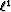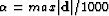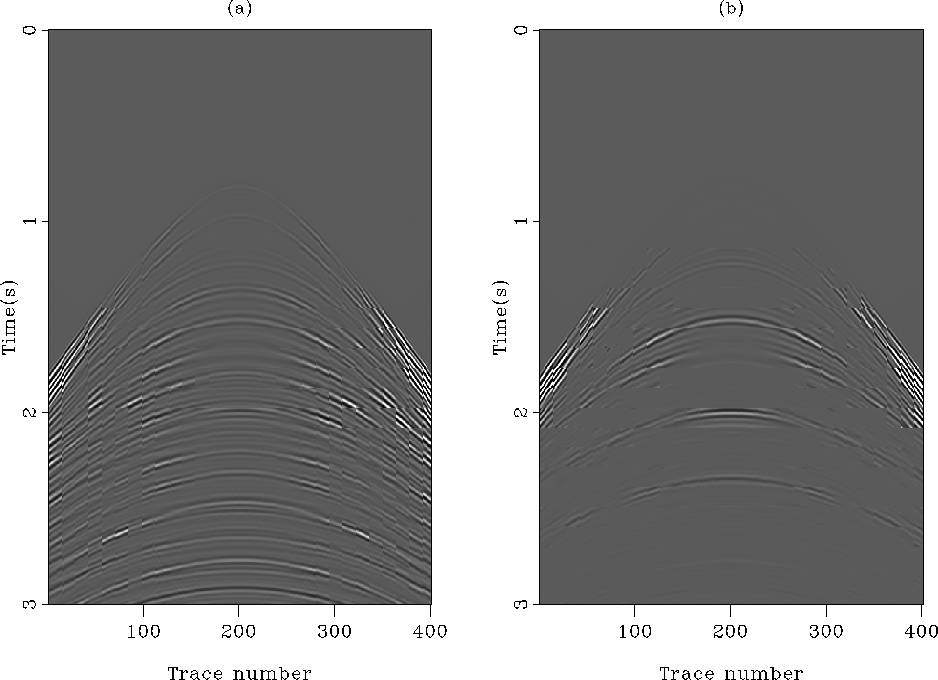Next: Poststack land data multiple Up: 2-D data example: attenuation Previous: Adaptive filtering with non-stationary

Figurea shows the estimated primaries when thenorm is used to compute the shaping filters. Figureb displays the estimated internal multiples. As expected, because of the local amplitude differences between the signal (primaries) and the noise (multiples), the adaptive subtraction fails and we retrieve the behavior explained in the preceding section with the 1-D example. Now, in Figure, we see the beneficial effects of thenorm. Figurea shows the estimated primaries and Figureb the estimated multiples. The noise subtracted matches very well the internal multiple model in Figureb, as anticipated. Note that with this dataset,had to be changed to.comp-diffL1L2
Figure 9
(a) The difference between the exact multiples (Figureb) and the subtracted multiples with thenorm. (b) The difference between the exact multiples (Figureb) and the subtracted multiples with thenorm.As a final comparison, Figuredisplays the difference between the internal-multiple model (Figureb) and the subtracted multiples with the two norms. Thenorm (Figureb) matches the multiple model much better than thenorm (Figurea).Next: Poststack land data multiple Up: 2-D data example: attenuation Previous: Adaptive filtering with non-stationary
Stanford Exploration Project
5/5/2005